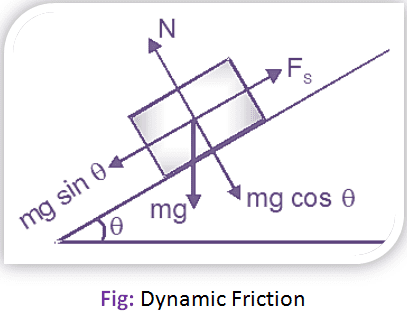# Different Types of Friction

## Types of Friction:

1. Static Friction: It is the friction, experienced by a body, when at rest. Static friction enters calculations as the Coefficient of Static Friction (µ), which is not a force in itself. The coefficient of static friction is used to find the Force of Static Friction (fs), which is a force that acts directly opposite the force being applied. The force of static friction is found by multiplying the coefficient of static by the normal force (FN).

∴ µs.FN = fs2. Dynamic Friction: It is the friction, experienced by a body, when in motion. The dynamic friction is also called kinetic friction and is less than the static friction.## Types of Dynamic Friction:

There are mainly three types of dynamic friction:

i. Sliding Friction: It is the friction, experienced by a body, when it slides over another body.

ii. Rolling Friction: It is the friction, experienced between the surfaces which has balls or rollers interposed between them.

iii. Pivot Friction: It is the friction, experienced by a body, due to the motion of rotation as in case of foot step bearings.